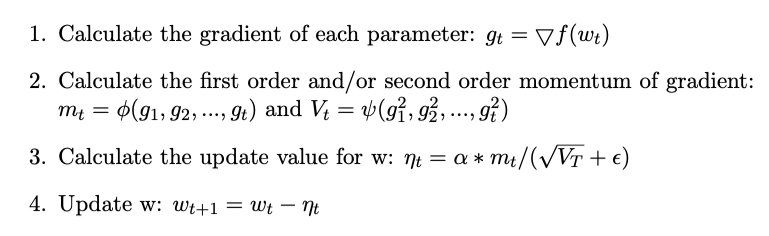# Overview of optimizers for DNN: when and how to choose which optimizer.

Original article can be found here (source): Deep Learning on Medium# Part 1: Review of optimization methods of DNN

## Section 1–1. Intuitive perspective of optimization

The goal of the optimization of DNN is to find the best parameters w to minimize the loss function f(w, x, y) subject to x, y, where x are the data and y are the labels. The gradient descent (GD) is the most frequently used method in Machine learning. In this method, we need another parameter called learning rate, α.

To sum up:

1. w, the parameters to be optimized
2. loss function f(w, x, y), we use f(w) bellow for simplification
3. learning rate: α

Now we start gradient descent, at each batch/step t: